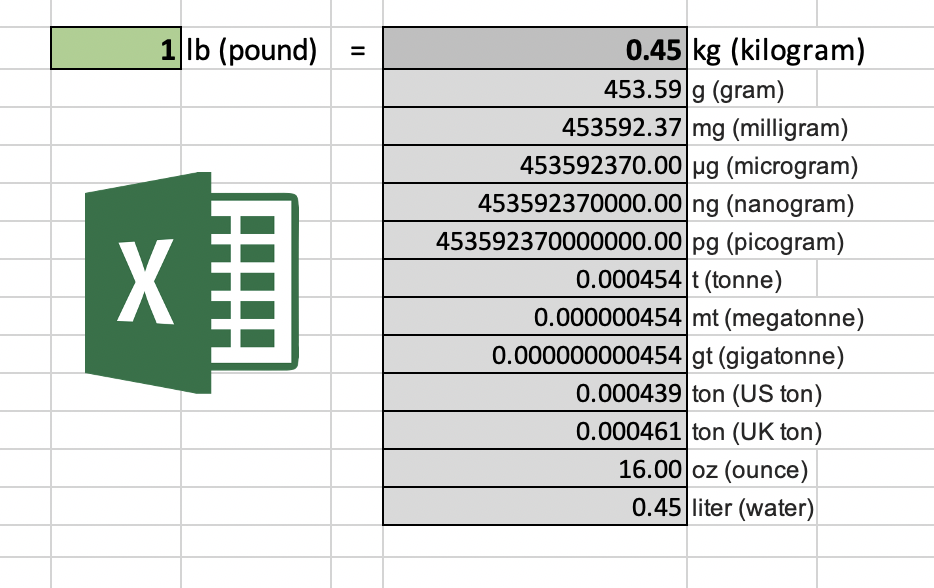# Pounds (lbs) to Kilograms (kg) Converter

Convert lbs to kg (Pounds to Kilograms). How many kilograms are pounds? How heavy are lbs in kg?

## Convert Pounds to Kilograms

=
Formula: Multiply lbs with 0.45359237

## How to Convert lbs to kg?

Converting pounds to kilograms is based on a simple formula of multiplying pounds by 0.45359237. To find out how many kilograms are in a pound, we can use a formula:

1 lbs * 0.45359237 kg/lbs = 0.45359237 kg

You may also wonder how many pounds are in a kilogram. We need to divide the kilograms with 0.45359237 to get the result:

1 kg / 0.45359237 kg/lbs = 2.2046226218488 lbs

## Popular lbs to kg Conversions Table

Pound Kilogram
1 lbs 0.45359237 kg
5 lbs 2.26796185 kg
10 lbs 4.5359237 kg
50 lbs 22.6796185 kg
100 lbs 45.359237 kg
200 lbs 90.718474 kg
300 lbs 136.077711 kg
400 lbs 181.436948 kg
500 lbs 226.796185 kg
1000 lbs 453.59237 kg
10000 lbs 4535.9237 kg

## Free Pounds to Kilograms Excel TemplateWe also have a free Microsoft Excel (.xlsx) template for Excel lovers. This template will convert lbs to kg and other common masses like tonnes and ounces.